# Selina Solutions Concise Maths Class 10 Chapter 17 Circles Exercise 17(A)

Fundamentals of circles and relation between its parts like segment, arc, chord are discussed in these exercise problems. The cyclic properties are also covered in this exercise. The Selina Solutions for Class 10 Maths is one valuable resource a student can have when solving problems of the book. It helps clear doubts instantly and improves problem-solving skills of the students. The Concise Selina Solutions for Class 10 Maths Chapter 17 Circles Exercise 17(A) PDF, is provided in the link given below.

## Selina Solutions Concise Maths Class 10 Chapter 17 Circles Exercise 17(A) Download PDF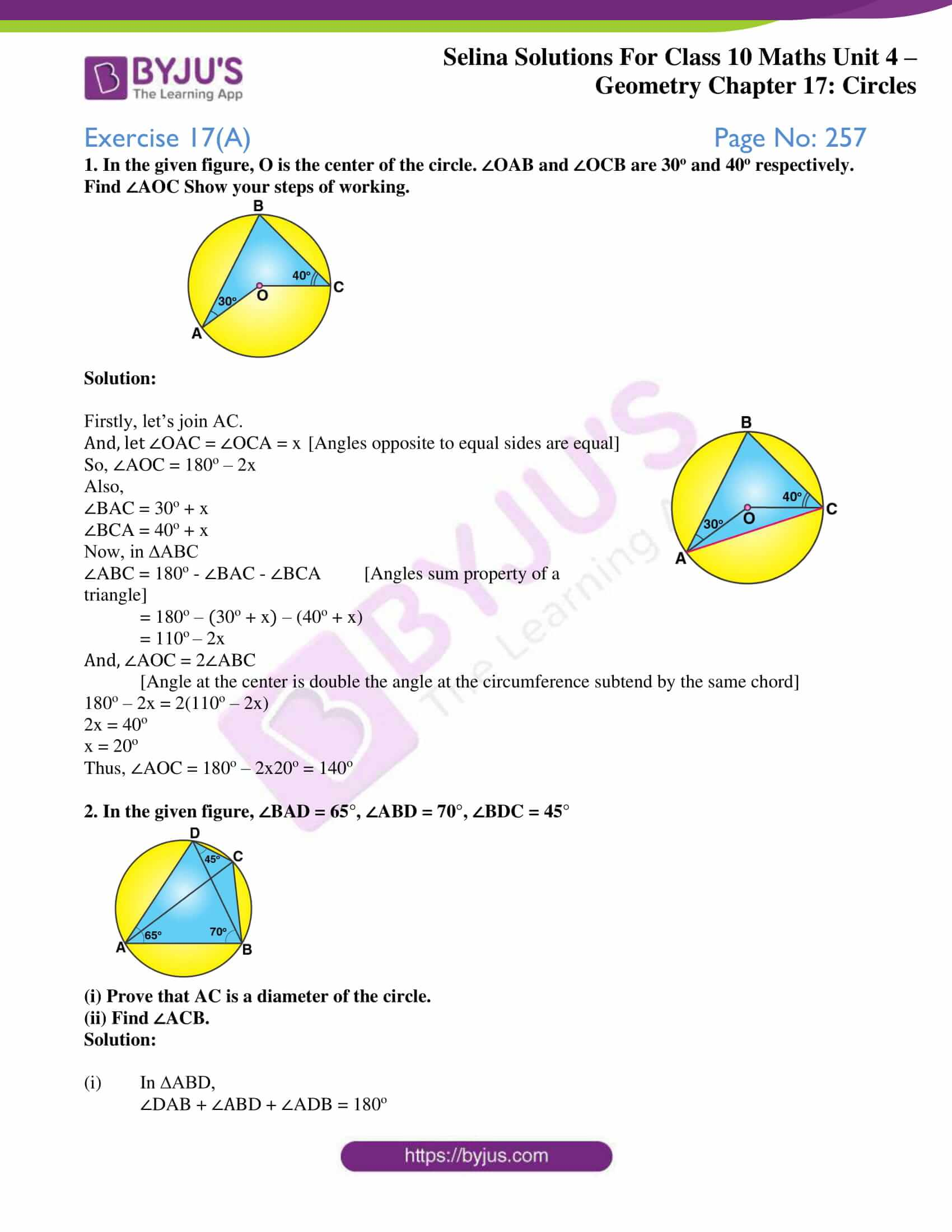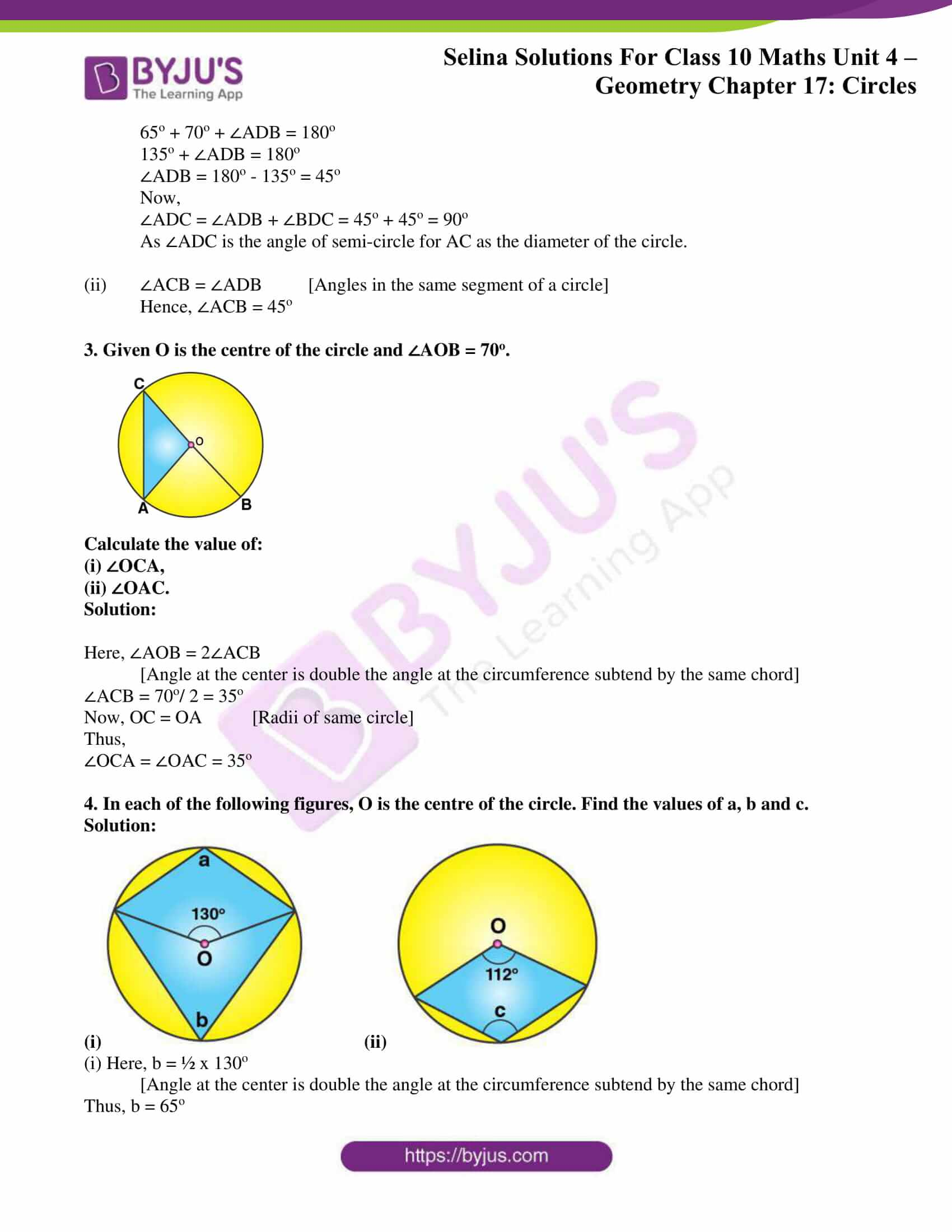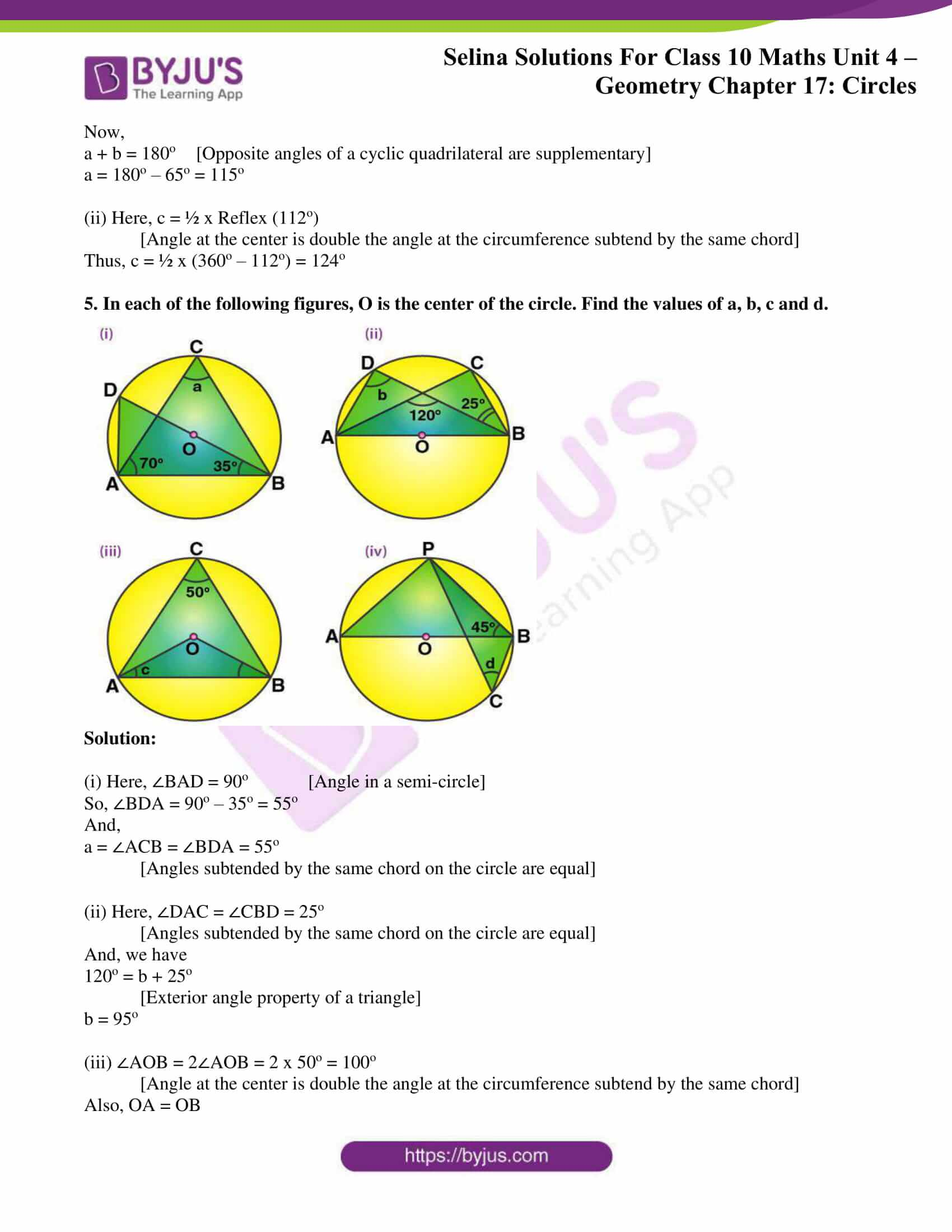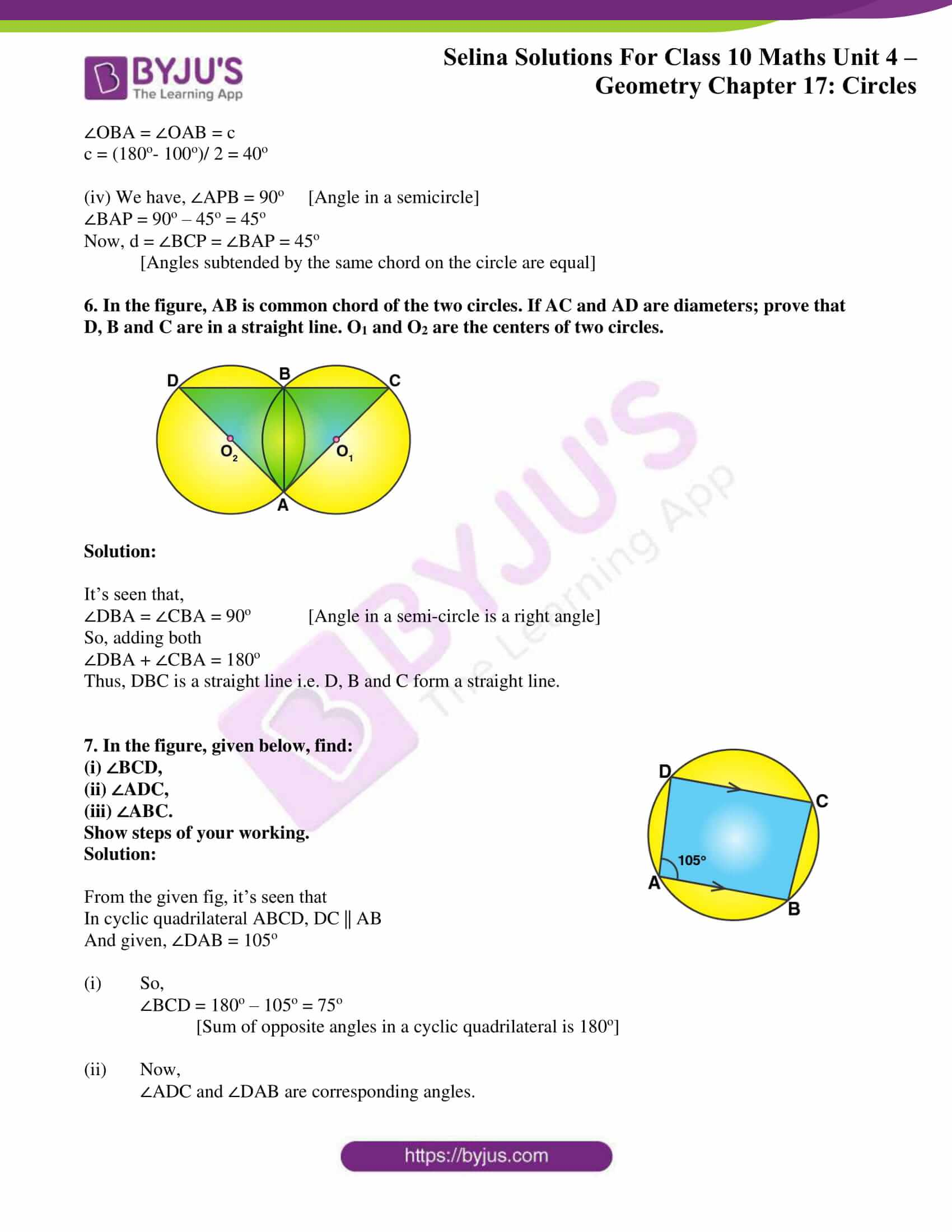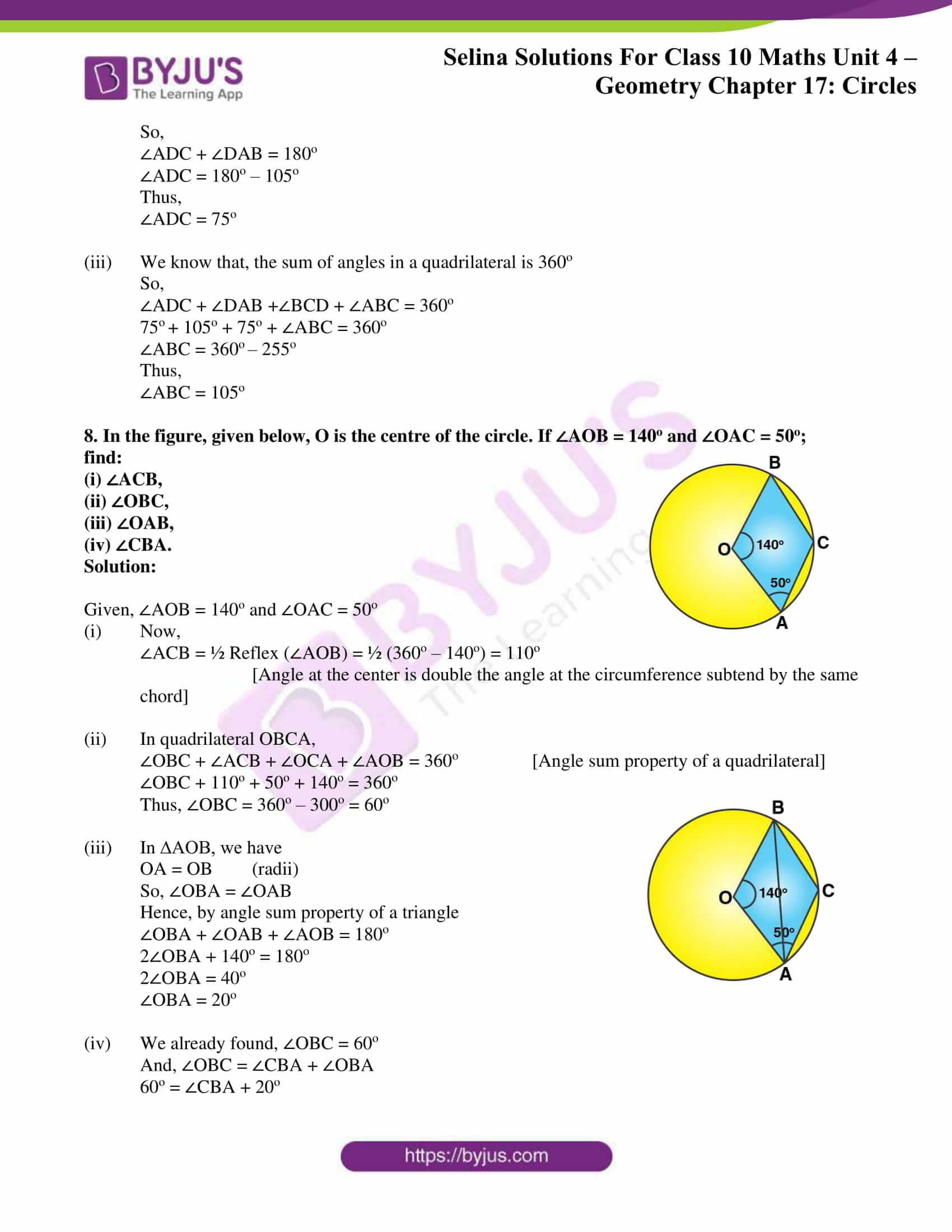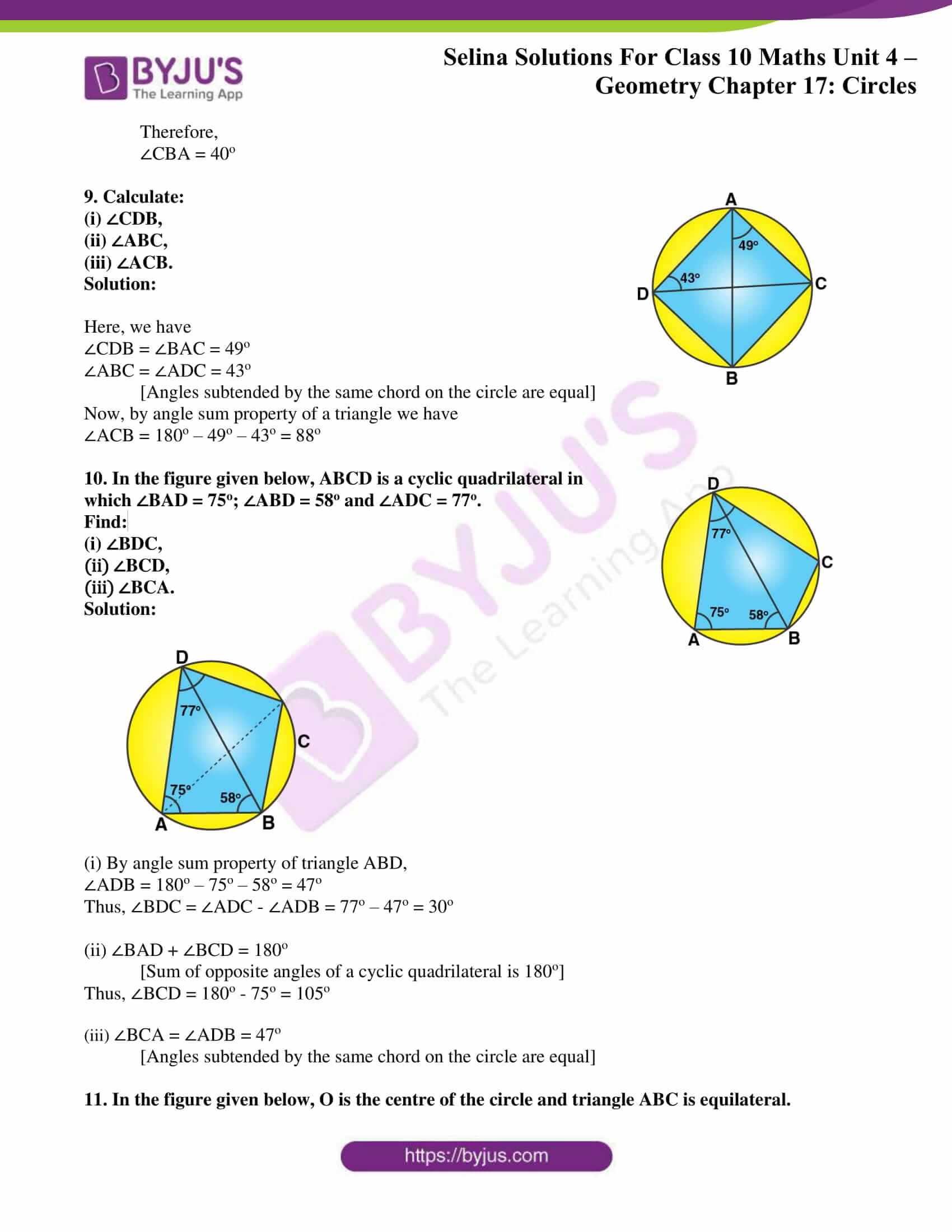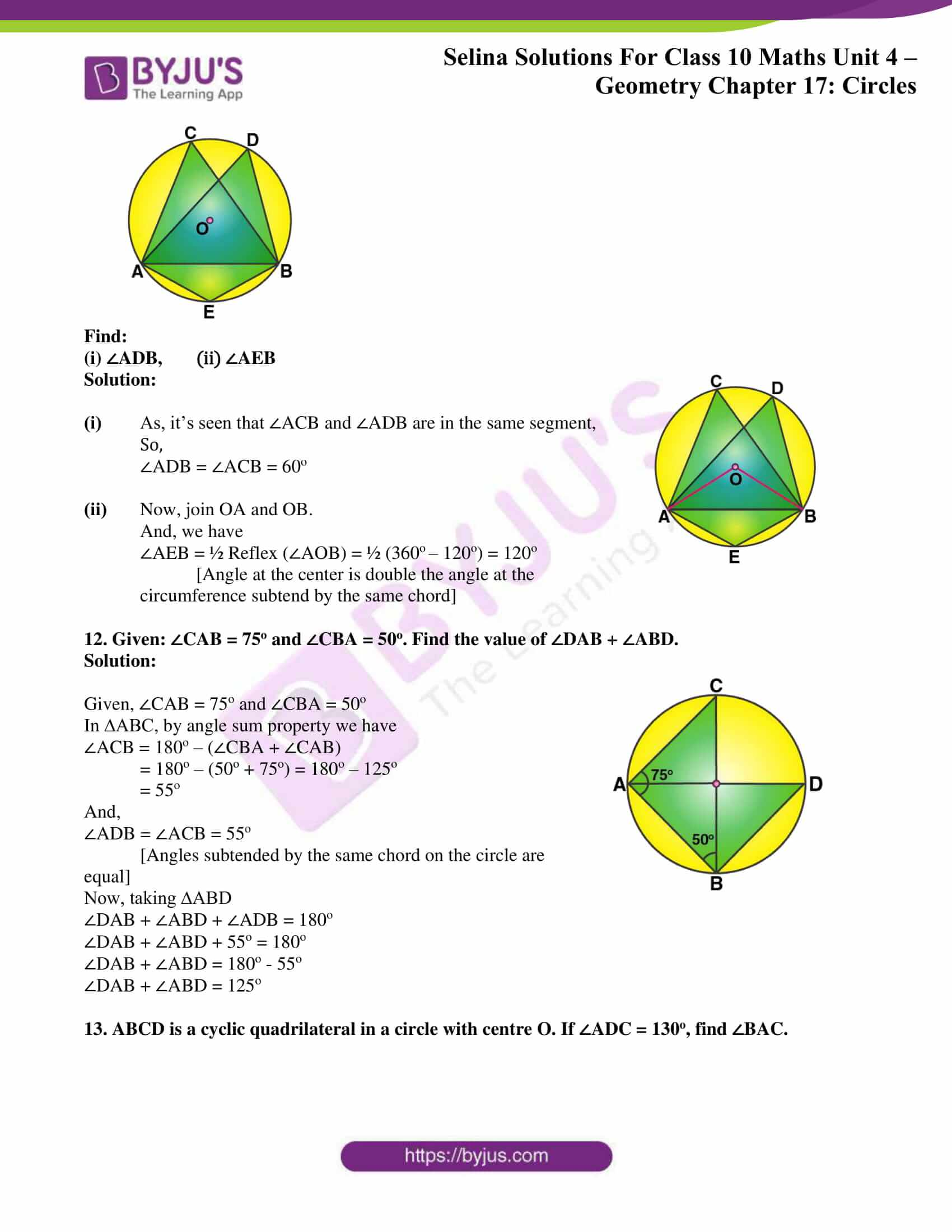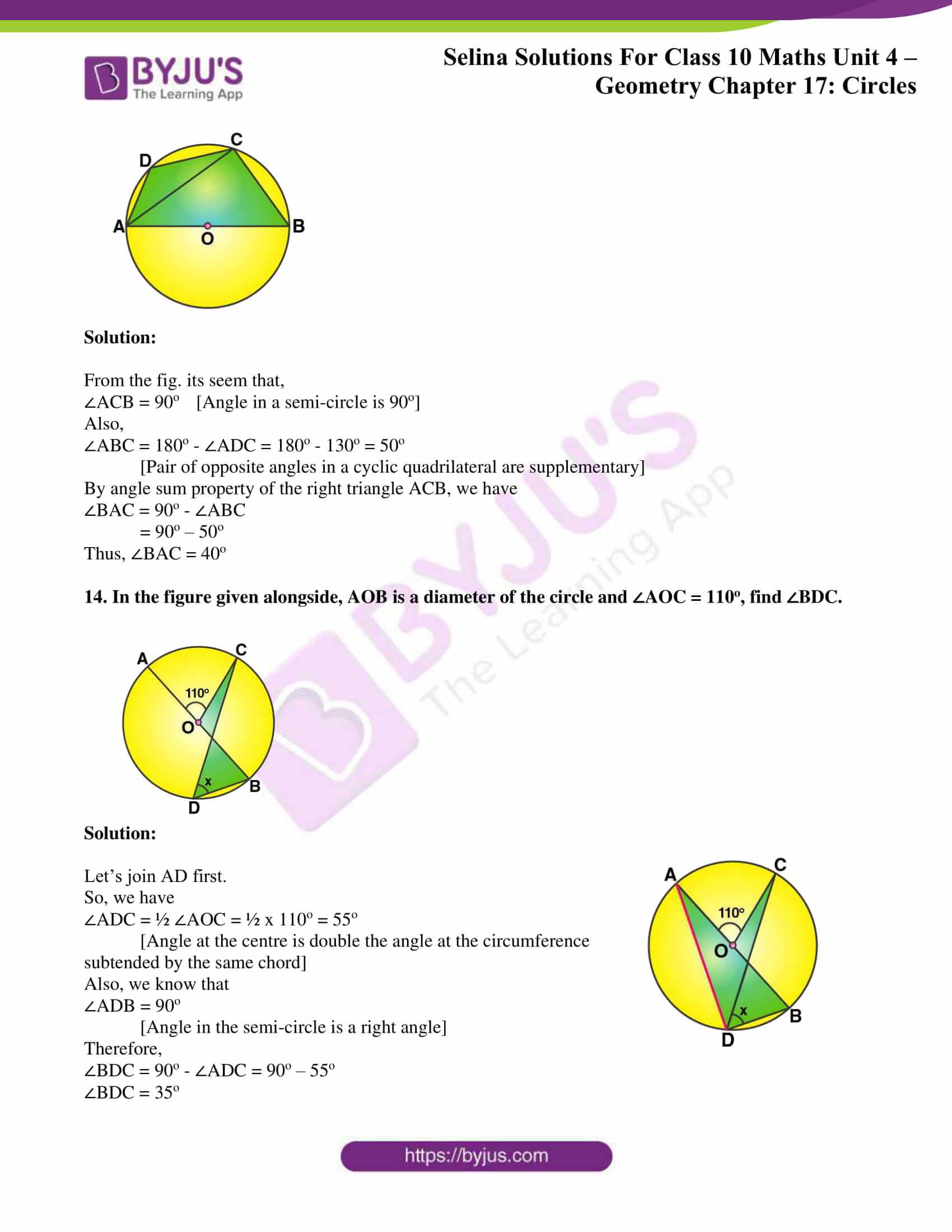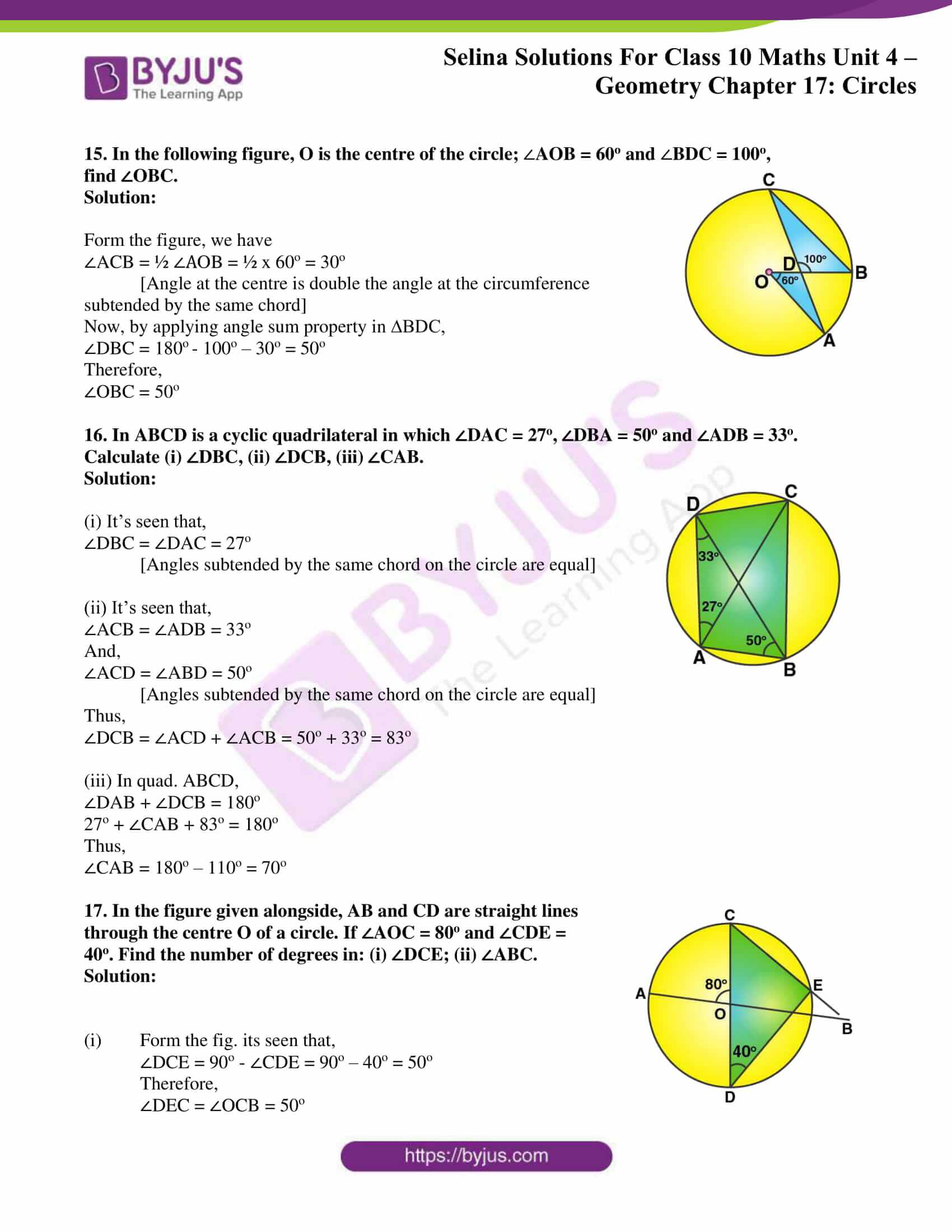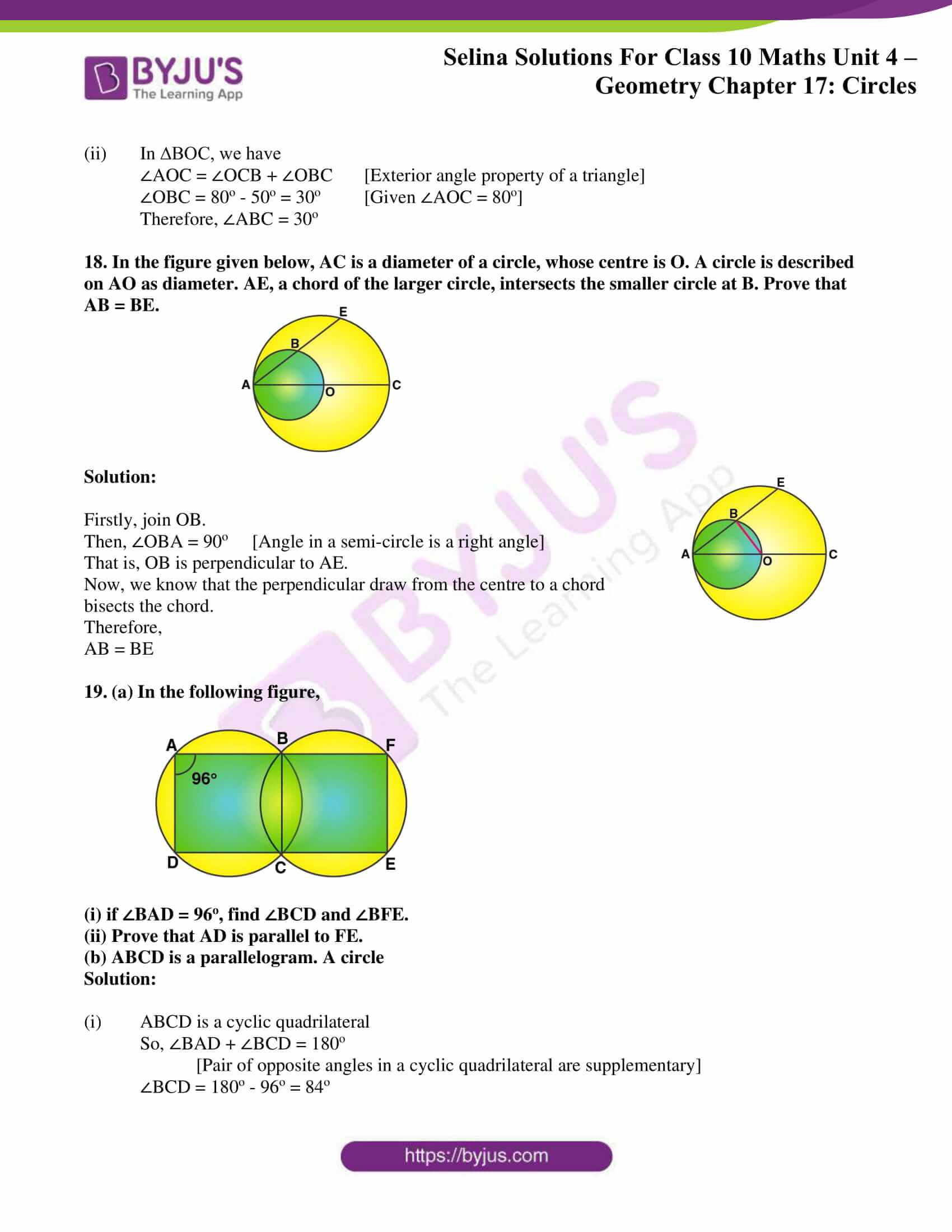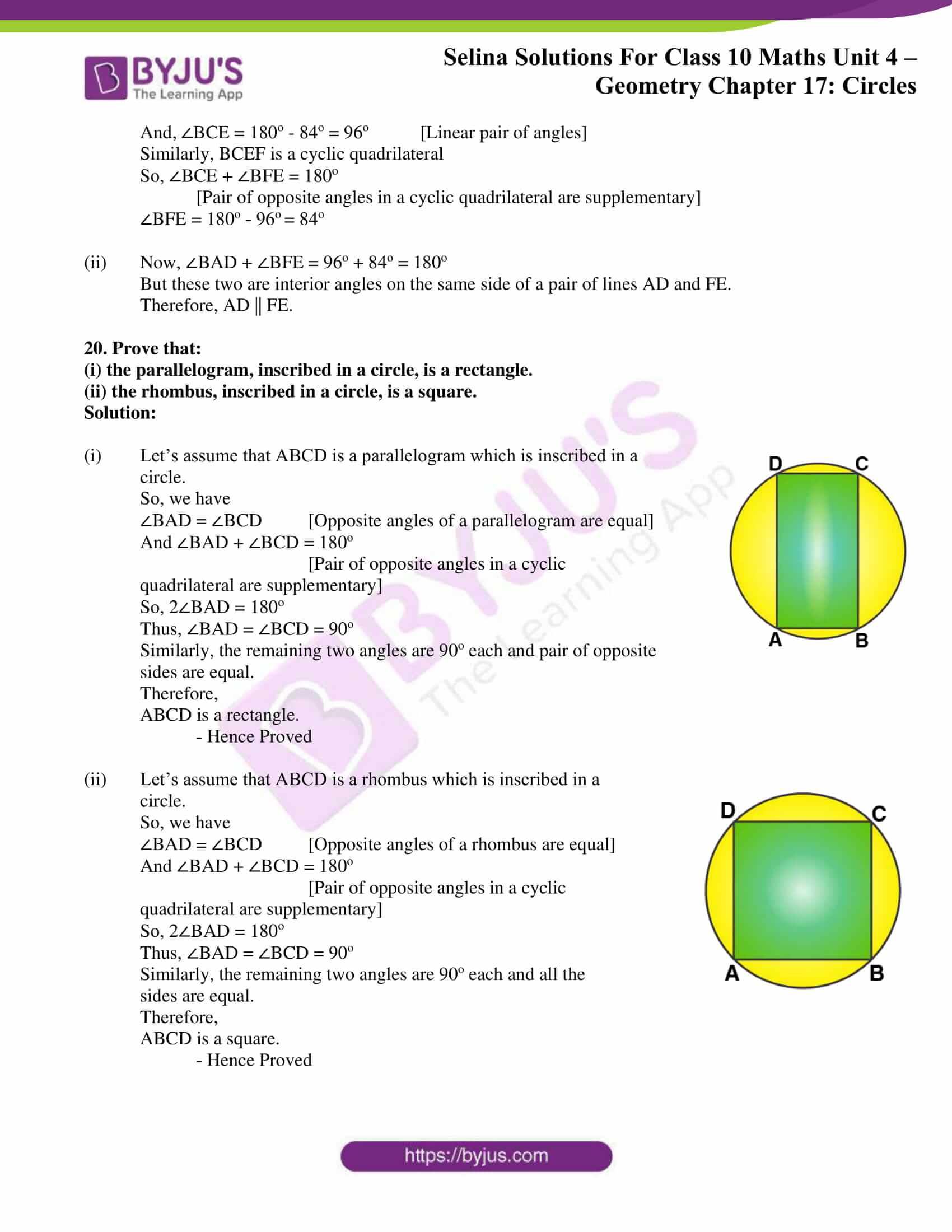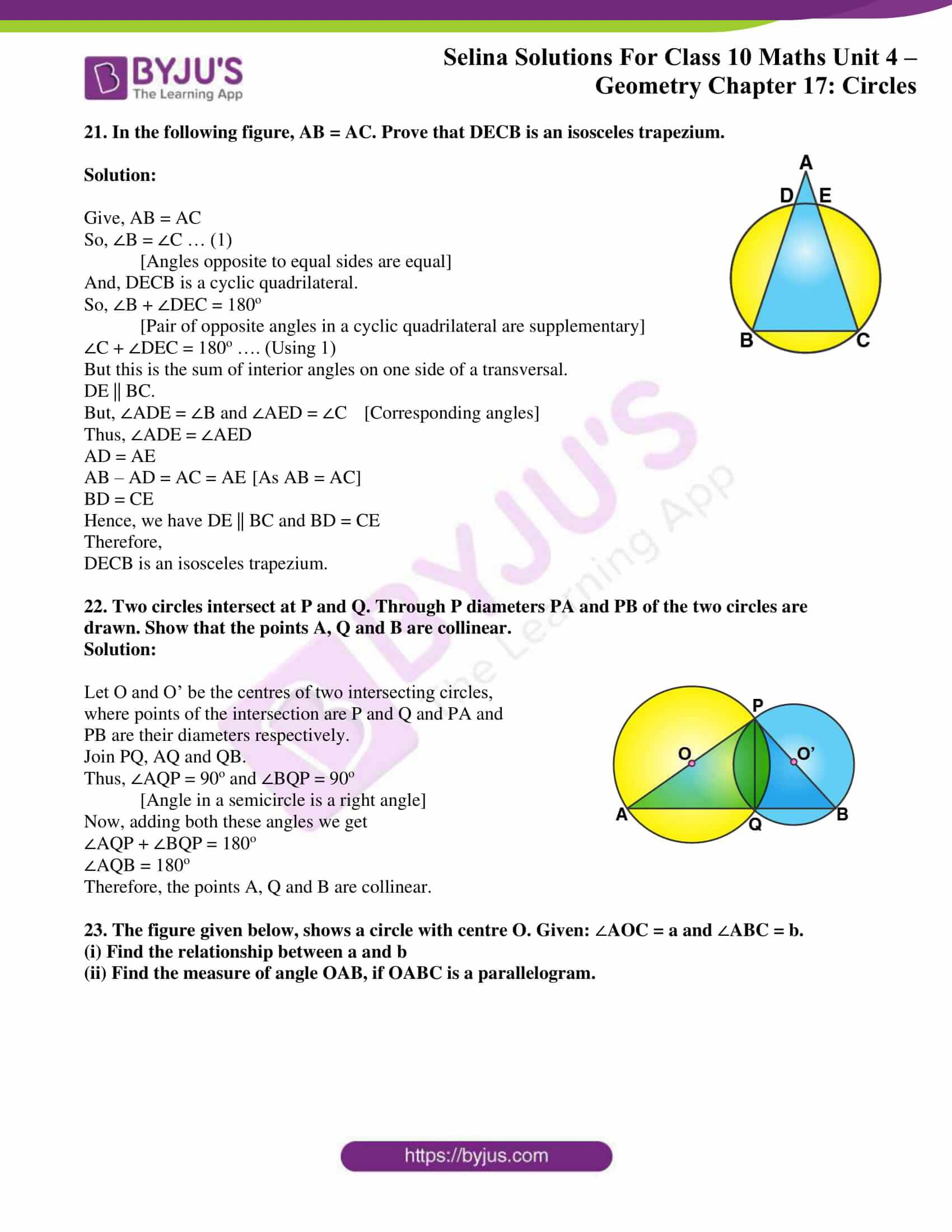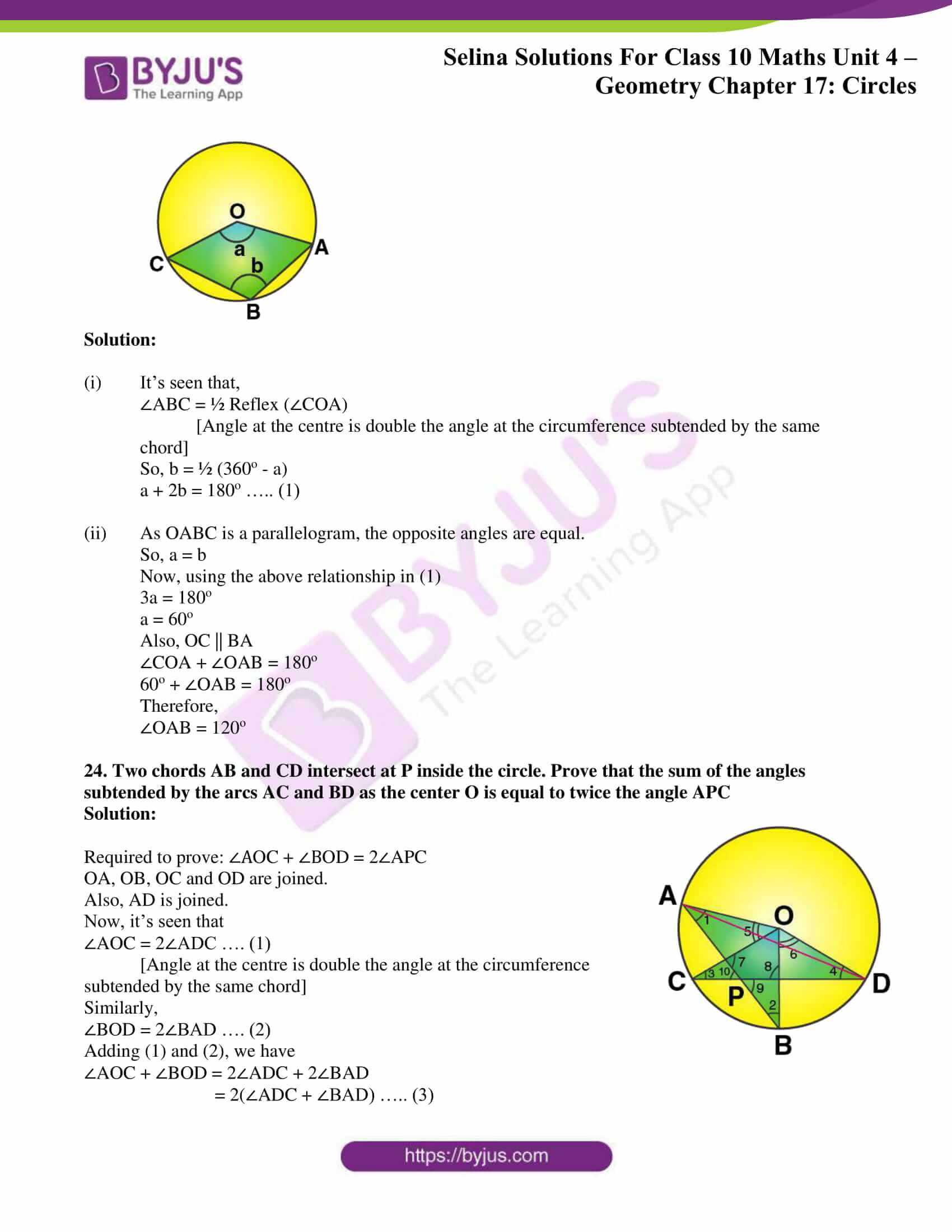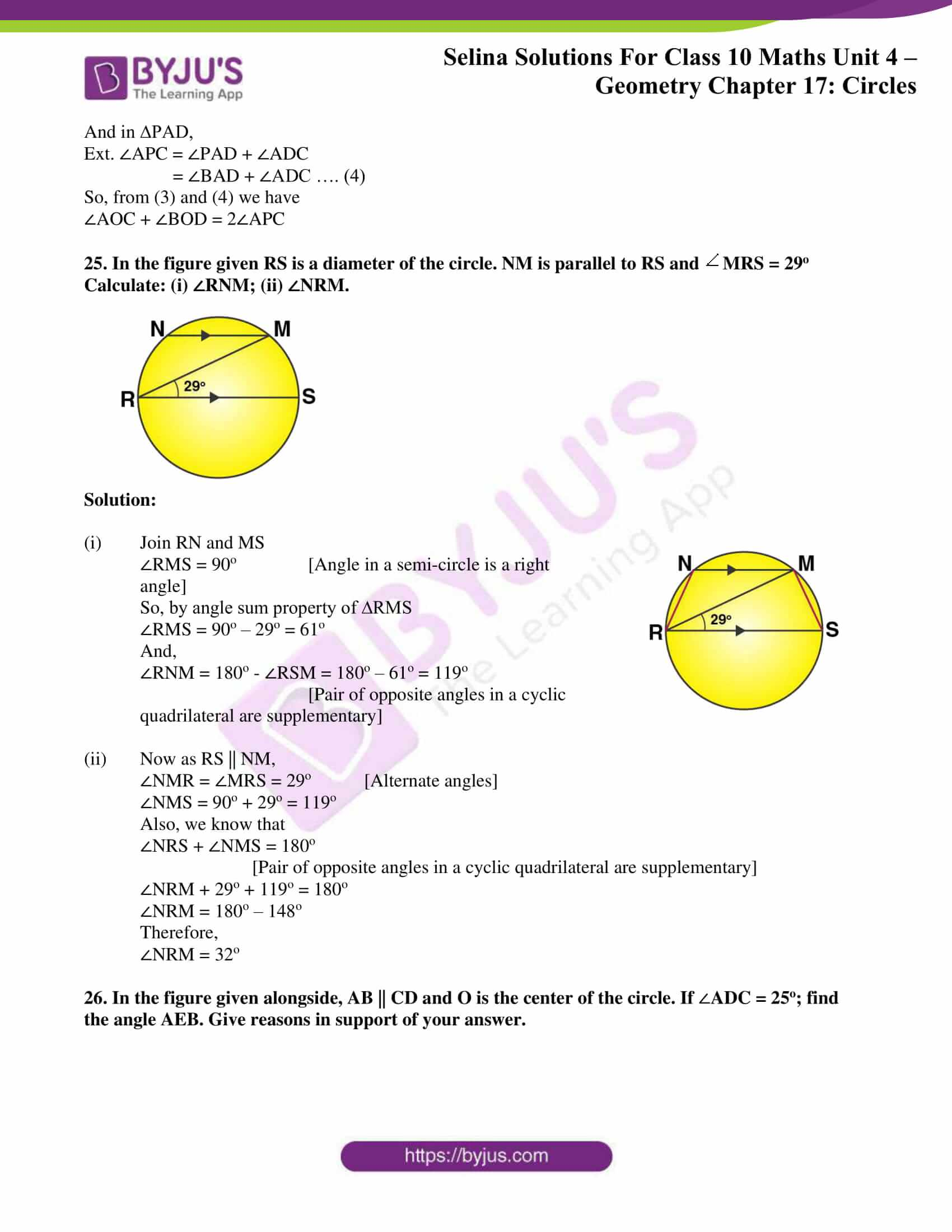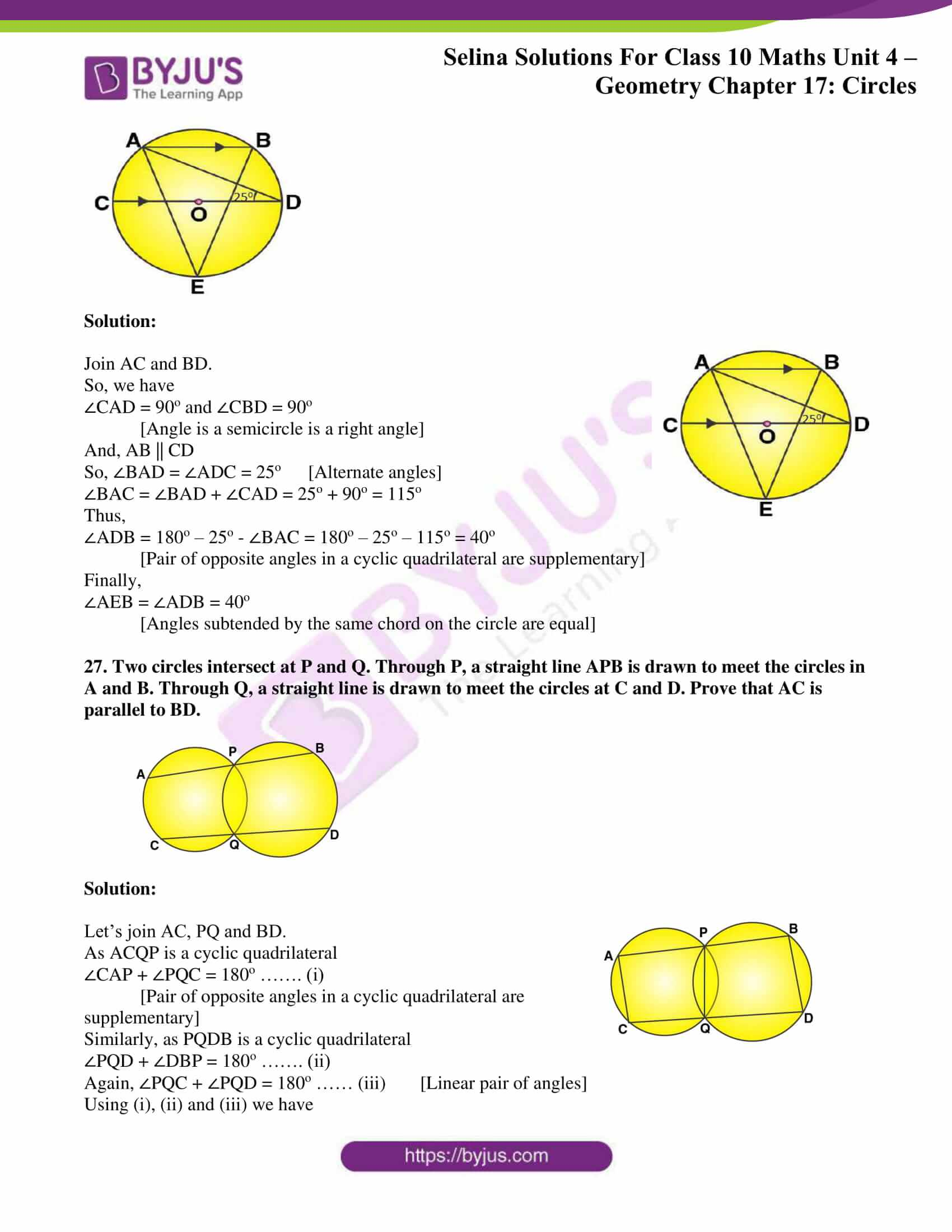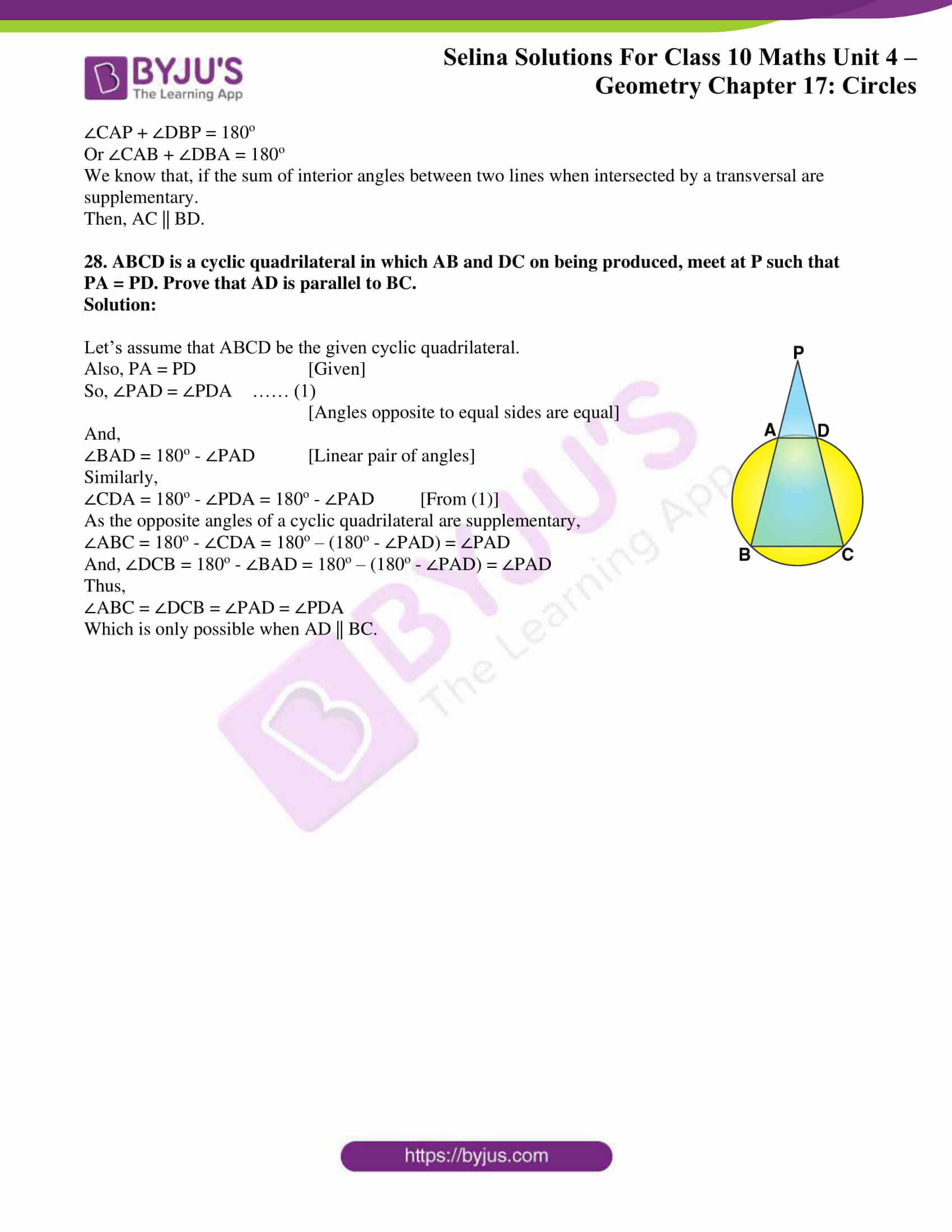### Access other exercises of Selina Solutions Concise Maths Class 10 Chapter 17 Circles

Exercise 17(B) Solutions

Exercise 17(C) Solutions

### Access Selina Solutions Concise Maths Class 10 Chapter 17 Circles Exercise 17(A)

1. In the given figure, O is the center of the circle. ∠OAB and ∠OCB are 30o and 40o respectively. Find ∠AOC Show your steps of working.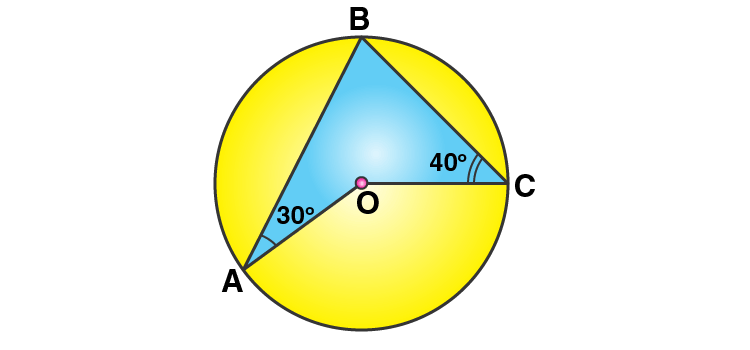Solution: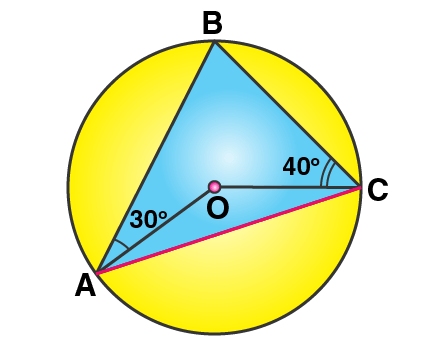Firstly, let’s join AC.

And, let ∠OAC = ∠OCA = x [Angles opposite to equal sides are equal]

So, ∠AOC = 180o – 2x

Also,

∠BAC = 30o + x

∠BCA = 40o + x

Now, in ∆ABC

∠ABC = 180o – ∠BAC – ∠BCA [Angles sum property of a triangle]

= 180o – (30o + x) – (40o + x)

= 110o – 2x

And, ∠AOC = 2∠ABC

[Angle at the center is double the angle at the circumference subtend by the same chord]

180o – 2x = 2(110o – 2x)

2x = 40o

x = 20o

Thus, ∠AOC = 180o – 2×20o = 140o

2. In the given figure, ∠BAD = 65°, ∠ABD = 70°, ∠BDC = 45°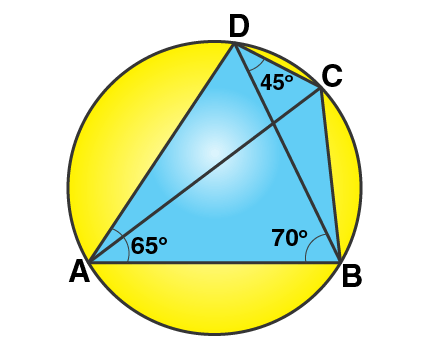(i) Prove that AC is a diameter of the circle.

(ii) Find ∠ACB.

Solution:

(i) In ∆ABD,

∠DAB + ∠ABD + ∠ADB = 180o

65o + 70o + ∠ADB = 180o

∠ADB = 180o – 135o = 45o

Now,

As ∠ADC is the angle of semi-circle for AC as the diameter of the circle.

(ii) ∠ACB = ∠ADB [Angles in the same segment of a circle]

Hence, ∠ACB = 45o

3. Given O is the centre of the circle and ∠AOB = 70o.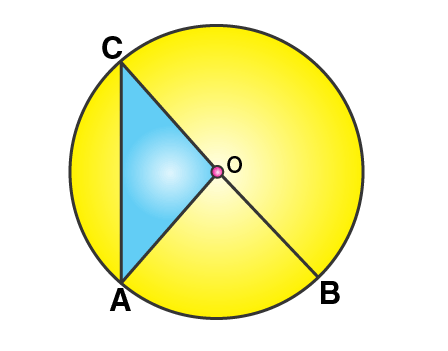Calculate the value of:

(i) ∠OCA,

(ii) ∠OAC.

Solution:

Here, ∠AOB = 2∠ACB

[Angle at the center is double the angle at the circumference subtend by the same chord]

∠ACB = 70o/ 2 = 35o

Now, OC = OA [Radii of same circle]

Thus,

∠OCA = ∠OAC = 35o

4. In each of the following figures, O is the centre of the circle. Find the values of a, b and c.

Solution:

(i)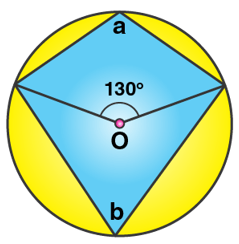(ii)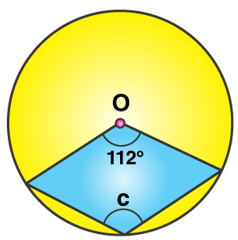(i) Here, b = ½ x 130o

[Angle at the center is double the angle at the circumference subtend by the same chord]

Thus, b = 65o

Now,

a + b = 180o [Opposite angles of a cyclic quadrilateral are supplementary]

a = 180o – 65o = 115o

(ii) Here, c = ½ x Reflex (112o)

[Angle at the center is double the angle at the circumference subtend by the same chord]

Thus, c = ½ x (360o – 112o) = 124o

5. In each of the following figures, O is the center of the circle. Find the values of a, b, c and d.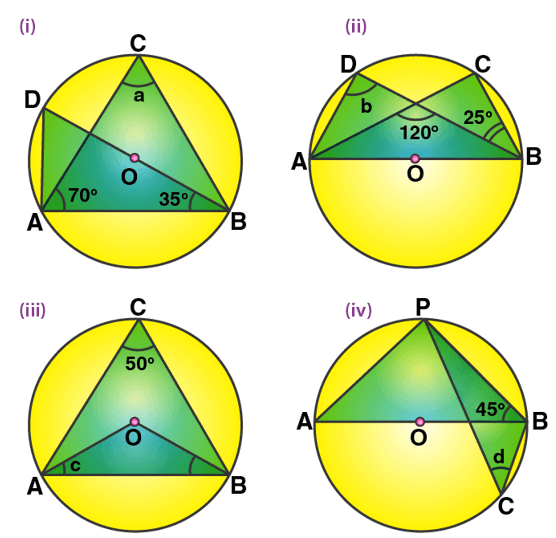Solution:

(i) Here, ∠BAD = 90o [Angle in a semi-circle]

So, ∠BDA = 90o – 35o = 55o

And,

a = ∠ACB = ∠BDA = 55o

[Angles subtended by the same chord on the circle are equal]

(ii) Here, ∠DAC = ∠CBD = 25o

[Angles subtended by the same chord on the circle are equal]

And, we have

120o = b + 25o

[Exterior angle property of a triangle]

b = 95o

(iii) ∠AOB = 2∠AOB = 2 x 50o = 100o

[Angle at the center is double the angle at the circumference subtend by the same chord]

Also, OA = OB

∠OBA = ∠OAB = c

c = (180o– 100o)/ 2 = 40o

(iv) We have, ∠APB = 90o [Angle in a semicircle]

∠BAP = 90o – 45o = 45o

Now, d = ∠BCP = ∠BAP = 45o

[Angles subtended by the same chord on the circle are equal]

6. In the figure, AB is common chord of the two circles. If AC and AD are diameters; prove that D, B and C are in a straight line. O1 and O2 are the centers of two circles.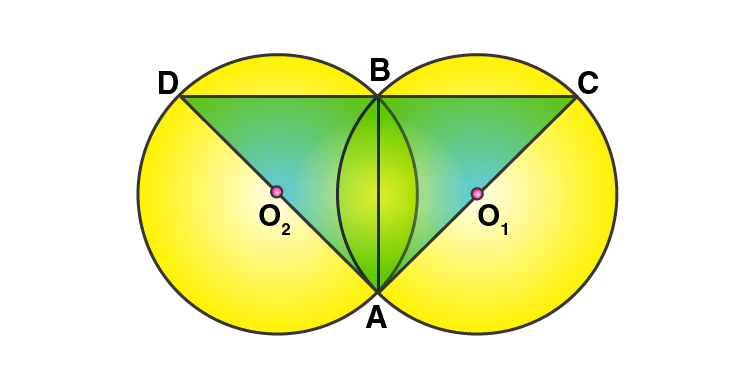Solution:

It’s seen that,

∠DBA = ∠CBA = 90o [Angle in a semi-circle is a right angle]

∠DBA + ∠CBA = 180o

Thus, DBC is a straight line i.e. D, B and C form a straight line.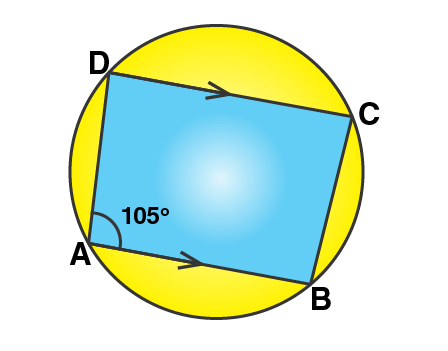7. In the figure, given below, find:

(i) ∠BCD,

(iii) ∠ABC.

Solution:

From the given fig, it’s seen that

In cyclic quadrilateral ABCD, DC || AB

And given, ∠DAB = 105o

(i) So,

∠BCD = 180o – 105o = 75o

[Sum of opposite angles in a cyclic quadrilateral is 180o]

(ii) Now,

∠ADC and ∠DAB are corresponding angles.

So,

Thus,

(iii) We know that, the sum of angles in a quadrilateral is 360o

So,

∠ADC + ∠DAB +∠BCD + ∠ABC = 360o

75o + 105o + 75o + ∠ABC = 360o

∠ABC = 360o – 255o

Thus,

∠ABC = 105o

8. In the figure, given below, O is the centre of the circle. If ∠AOB = 140o and ∠OAC = 50o;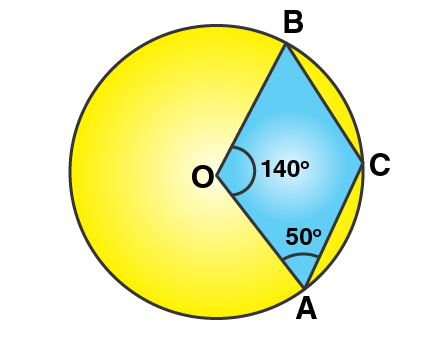find:

(i) ∠ACB,

(ii) ∠OBC,

(iii) ∠OAB,

(iv) ∠CBA.

Solution:

Given, ∠AOB = 140o and ∠OAC = 50o

(i) Now,

∠ACB = ½ Reflex (∠AOB) = ½ (360o – 140o) = 110o

[Angle at the center is double the angle at the circumference subtend by the same chord]

∠OBC + ∠ACB + ∠OCA + ∠AOB = 360o [Angle sum property of a quadrilateral]

∠OBC + 110o + 50o + 140o = 360o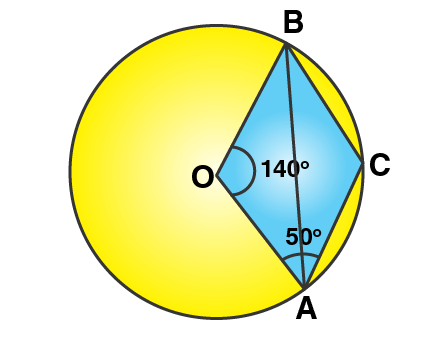Thus, ∠OBC = 360o – 300o = 60o

(iii) In ∆AOB, we have

So, ∠OBA = ∠OAB

Hence, by angle sum property of a triangle

∠OBA + ∠OAB + ∠AOB = 180o

2∠OBA + 140o = 180o

2∠OBA = 40o

∠OBA = 20o

(iv) We already found, ∠OBC = 60o

And, ∠OBC = ∠CBA + ∠OBA

60o = ∠CBA + 20o

Therefore,

∠CBA = 40o

9. Calculate: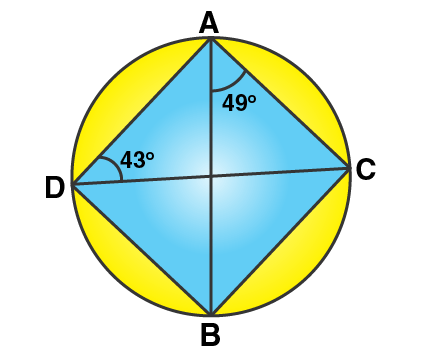(i) ∠CDB,

(ii) ∠ABC,

(iii) ∠ACB.

Solution:

Here, we have

∠CDB = ∠BAC = 49o

[Angles subtended by the same chord on the circle are equal]

Now, by angle sum property of a triangle we have

∠ACB = 180o – 49o – 43o = 88o

10. In the figure given below, ABCD is a cyclic quadrilateral in which ∠BAD = 75o; ∠ABD = 58o and ∠ADC = 77o.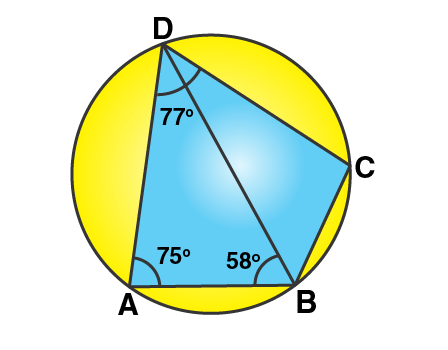Find:

(i) ∠BDC,

(ii) ∠BCD,

(iii) ∠BCA.

Solution: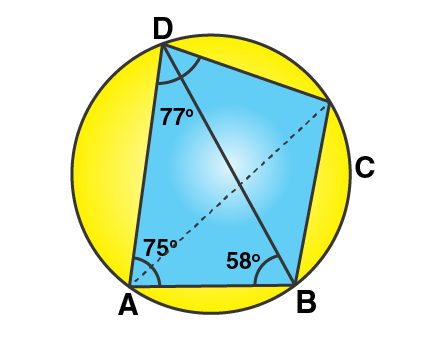(i) By angle sum property of triangle ABD,

∠ADB = 180o – 75o – 58o = 47o

Thus, ∠BDC = ∠ADC – ∠ADB = 77o – 47o = 30o

(ii) ∠BAD + ∠BCD = 180o

[Sum of opposite angles of a cyclic quadrilateral is 180o]

Thus, ∠BCD = 180o – 75o = 105o

(iii) ∠BCA = ∠ADB = 47o

[Angles subtended by the same chord on the circle are equal]

11. In the figure given below, O is the centre of the circle and triangle ABC is equilateral.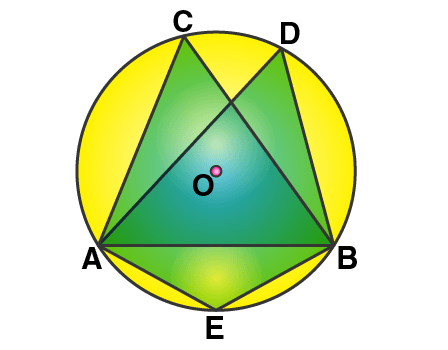Find: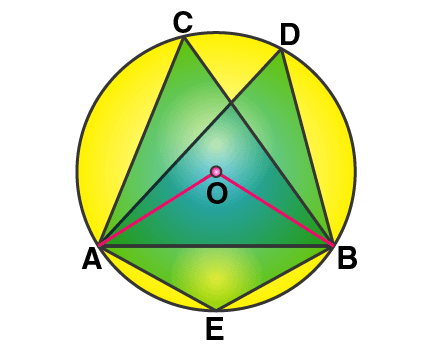Solution:

(i) As, it’s seen that ∠ACB and ∠ADB are in the same segment,

So,

(ii) Now, join OA and OB.

And, we have

∠AEB = ½ Reflex (∠AOB) = ½ (360o – 120o) = 120o

[Angle at the center is double the angle at the circumference subtend by the same chord]

12. Given: ∠CAB = 75o and ∠CBA = 50o. Find the value of ∠DAB + ∠ABD.

Solution: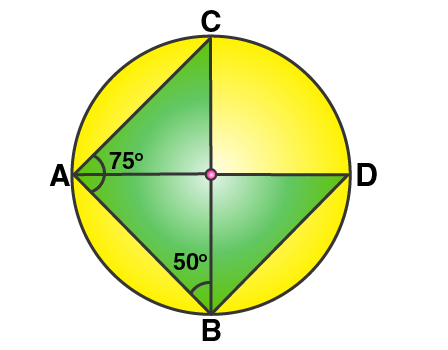Given, ∠CAB = 75o and ∠CBA = 50o

In ∆ABC, by angle sum property we have

∠ACB = 180o – (∠CBA + ∠CAB)

= 180o – (50o + 75o) = 180o – 125o

= 55o

And,

[Angles subtended by the same chord on the circle are equal]

Now, taking ∆ABD

∠DAB + ∠ABD + ∠ADB = 180o

∠DAB + ∠ABD + 55o = 180o

∠DAB + ∠ABD = 180o – 55o

∠DAB + ∠ABD = 125o

13. ABCD is a cyclic quadrilateral in a circle with centre O. If ∠ADC = 130o, find ∠BAC.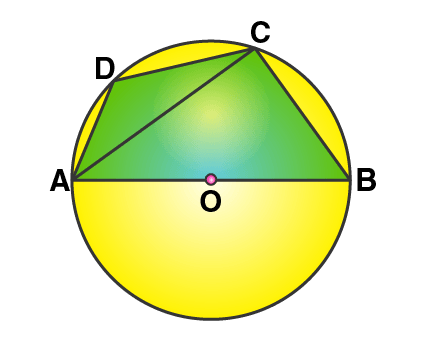Solution:

From the fig. its seem that,

∠ACB = 90o [Angle in a semi-circle is 90o]

Also,

∠ABC = 180o – ∠ADC = 180o – 130o = 50o

[Pair of opposite angles in a cyclic quadrilateral are supplementary]

By angle sum property of the right triangle ACB, we have

∠BAC = 90o – ∠ABC

= 90o – 50o

Thus, ∠BAC = 40o

14. In the figure given alongside, AOB is a diameter of the circle and ∠AOC = 110o, find ∠BDC.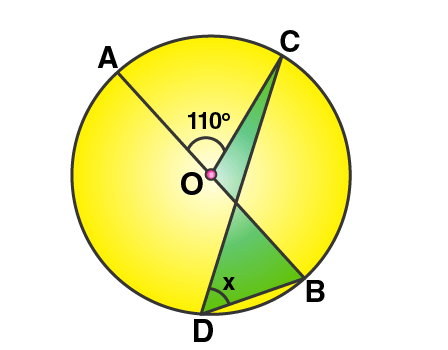Solution: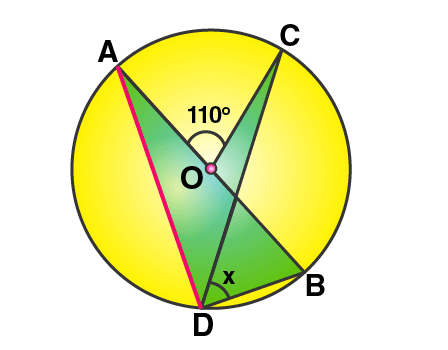So, we have

∠ADC = ½ ∠AOC = ½ x 110o = 55o

[Angle at the centre is double the angle at the circumference subtended by the same chord]

Also, we know that

[Angle in the semi-circle is a right angle]

Therefore,

∠BDC = 90o – ∠ADC = 90o – 55o

∠BDC = 35o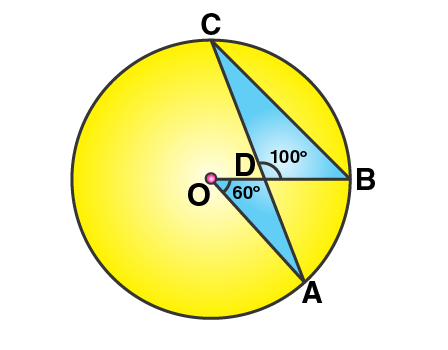15. In the following figure, O is the centre of the circle;AOB = 60o and BDC = 100o, find ∠OBC.

Solution:

Form the figure, we have

∠ACB = ½ ∠AOB = ½ x 60o = 30o

[Angle at the centre is double the angle at the circumference subtended by the same chord]

Now, by applying angle sum property in ∆BDC,

∠DBC = 180o – 100o – 30o = 50o

Therefore,

∠OBC = 50o

16. In ABCD is a cyclic quadrilateral in which ∠DAC = 27o, ∠DBA = 50o and ∠ADB = 33o. Calculate (i) ∠DBC, (ii) ∠DCB, (iii) ∠CAB.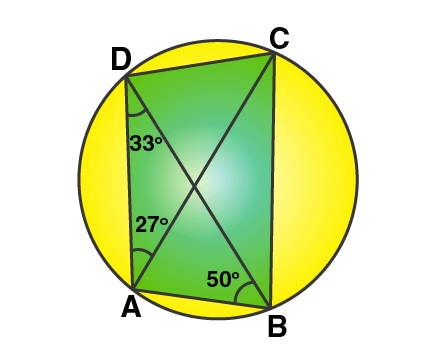Solution:

(i) It’s seen that,

∠DBC = ∠DAC = 27o

[Angles subtended by the same chord on the circle are equal]

(ii) It’s seen that,

And,

∠ACD = ∠ABD = 50o

[Angles subtended by the same chord on the circle are equal]

Thus,

∠DCB = ∠ACD + ∠ACB = 50o + 33o = 83o

∠DAB + ∠DCB = 180o

27o + ∠CAB + 83o = 180o

Thus,

∠CAB = 180o – 110o = 70o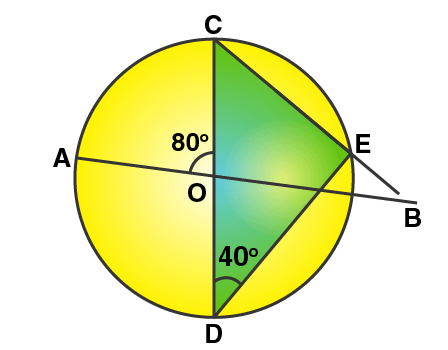17. In the figure given alongside, AB and CD are straight lines through the centre O of a circle. If ∠AOC = 80o and ∠CDE = 40o. Find the number of degrees in: (i) ∠DCE; (ii) ∠ABC.

Solution:

(i) Form the fig. its seen that,

∠DCE = 90o – ∠CDE = 90o – 40o = 50o

Therefore,

∠DEC = ∠OCB = 50o

(ii) In ∆BOC, we have

∠AOC = ∠OCB + ∠OBC [Exterior angle property of a triangle]

∠OBC = 80o – 50o = 30o  [Given ∠AOC = 80o]

Therefore, ∠ABC = 30o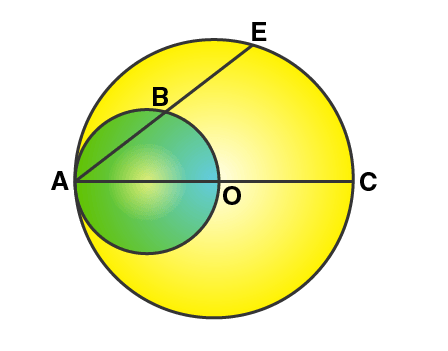18. In the figure given below, AC is a diameter of a circle, whose centre is O. A circle is described on AO as diameter. AE, a chord of the larger circle, intersects the smaller circle at B. Prove that AB = BE.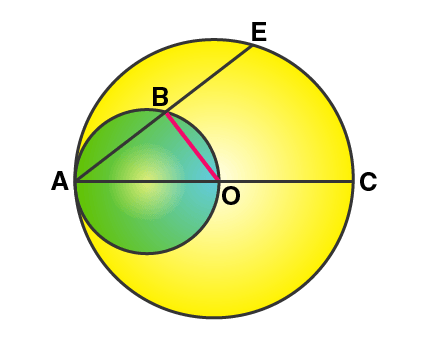Solution:

Firstly, join OB.

Then, ∠OBA = 90o [Angle in a semi-circle is a right angle]

That is, OB is perpendicular to AE.

Now, we know that the perpendicular draw from the centre to a chord bisects the chord.

Therefore,

AB = BE

19. (a) In the following figure,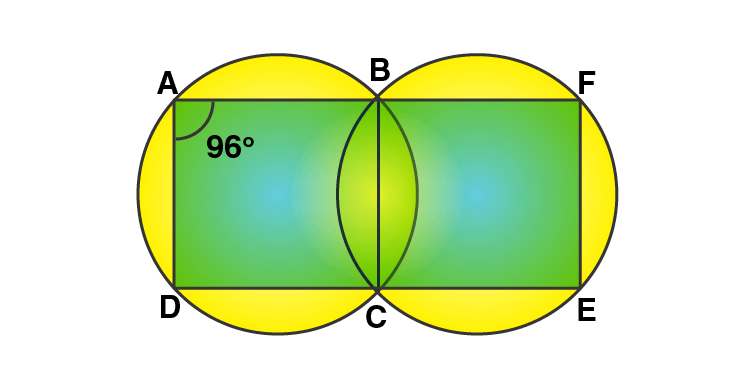(i) if ∠BAD = 96o, find ∠BCD and ∠BFE.

(ii) Prove that AD is parallel to FE.

(b) ABCD is a parallelogram. A circle

Solution:

(i) ABCD is a cyclic quadrilateral

So, ∠BAD + ∠BCD = 180o

[Pair of opposite angles in a cyclic quadrilateral are supplementary]

∠BCD = 180o – 96o = 84o

And, ∠BCE = 180o – 84o = 96o [Linear pair of angles]

Similarly, BCEF is a cyclic quadrilateral

So, ∠BCE + ∠BFE = 180o

[Pair of opposite angles in a cyclic quadrilateral are supplementary]

∠BFE = 180o – 96o = 84o

(ii) Now, ∠BAD + ∠BFE = 96o + 84o = 180o

But these two are interior angles on the same side of a pair of lines AD and FE.

20. Prove that:

(i) the parallelogram, inscribed in a circle, is a rectangle.

(ii) the rhombus, inscribed in a circle, is a square.

Solution: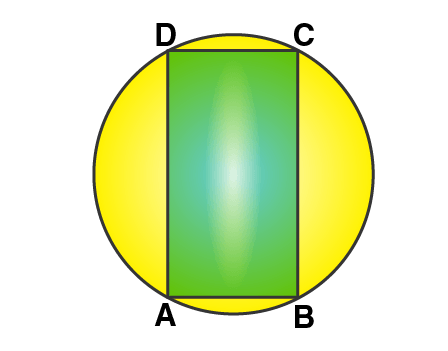(i) Let’s assume that ABCD is a parallelogram which is inscribed in a circle.

So, we have

∠BAD = ∠BCD [Opposite angles of a parallelogram are equal]

And ∠BAD + ∠BCD = 180o

[Pair of opposite angles in a cyclic quadrilateral are supplementary]

Thus, ∠BAD = ∠BCD = 90o

Similarly, the remaining two angles are 90o each and pair of opposite sides are equal.

Therefore,

ABCD is a rectangle.

– Hence Proved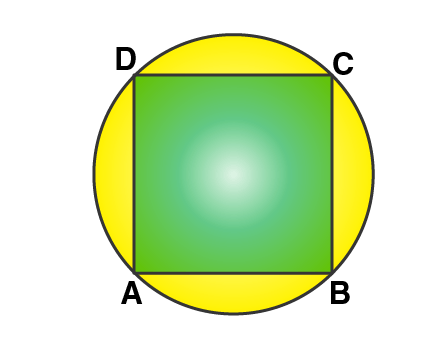(ii) Let’s assume that ABCD is a rhombus which is inscribed in a circle.

So, we have

∠BAD = ∠BCD [Opposite angles of a rhombus are equal]

And ∠BAD + ∠BCD = 180o

[Pair of opposite angles in a cyclic quadrilateral are supplementary]

Thus, ∠BAD = ∠BCD = 90o

Similarly, the remaining two angles are 90o each and all the sides are equal.

Therefore,

ABCD is a square.

– Hence Proved

21. In the following figure, AB = AC. Prove that DECB is an isosceles trapezium.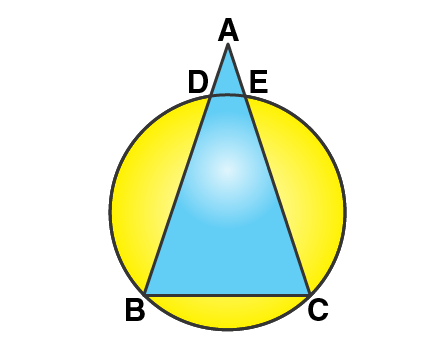Solution:

Give, AB = AC

So, ∠B = ∠C … (1)

[Angles opposite to equal sides are equal]

And, DECB is a cyclic quadrilateral.

So, ∠B + ∠DEC = 180o

[Pair of opposite angles in a cyclic quadrilateral are supplementary]

∠C + ∠DEC = 180o …. (Using 1)

But this is the sum of interior angles on one side of a transversal.

DE || BC.

But, ∠ADE = ∠B and ∠AED = ∠C [Corresponding angles]

AB – AD = AC = AE [As AB = AC]

BD = CE

Hence, we have DE || BC and BD = CE

Therefore,

DECB is an isosceles trapezium.

22. Two circles intersect at P and Q. Through P diameters PA and PB of the two circles are drawn. Show that the points A, Q and B are collinear.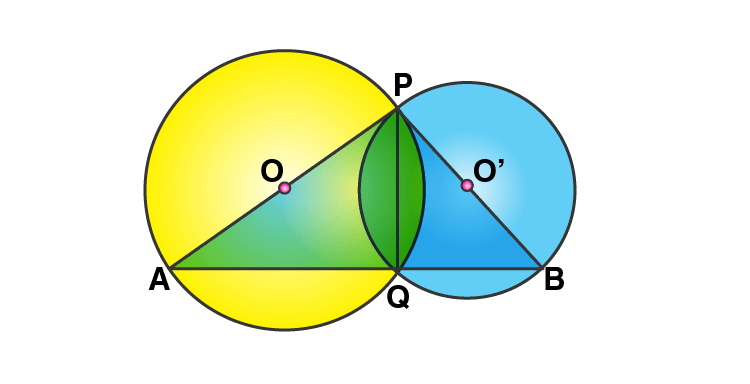Solution:

Let O and O’ be the centres of two intersecting circles, where points of the intersection are P and Q and PA and PB are their diameters respectively.

Join PQ, AQ and QB.

Thus, ∠AQP = 90o and ∠BQP = 90o

[Angle in a semicircle is a right angle]

Now, adding both these angles we get

∠AQP + ∠BQP = 180o

∠AQB = 180o

Therefore, the points A, Q and B are collinear.

23. The figure given below, shows a circle with centre O. Given: AOC = a and ABC = b.

(i) Find the relationship between a and b

(ii) Find the measure of angle OAB, if OABC is a parallelogram.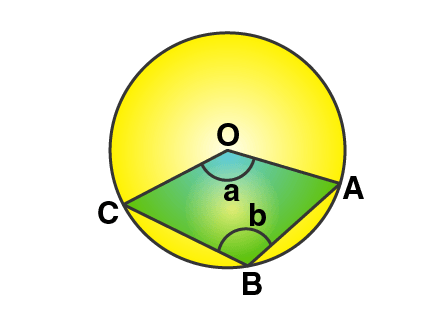Solution:

(i) It’s seen that,

∠ABC = ½ Reflex (∠COA)

[Angle at the centre is double the angle at the circumference subtended by the same chord]

So, b = ½ (360o – a)

a + 2b = 180o ….. (1)

(ii) As OABC is a parallelogram, the opposite angles are equal.

So, a = b

Now, using the above relationship in (1)

3a = 180o

a = 60o

Also, OC || BA

∠COA + ∠OAB = 180o

60o + ∠OAB = 180o

Therefore,

∠OAB = 120o

24. Two chords AB and CD intersect at P inside the circle. Prove that the sum of the angles subtended by the arcs AC and BD as the center O is equal to twice the angle APC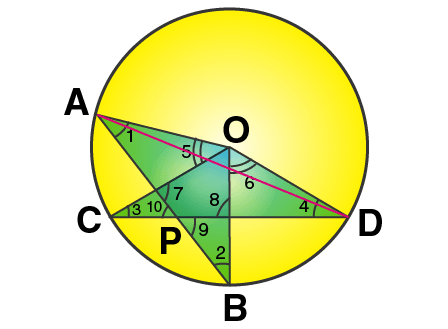Solution:

Required to prove: ∠AOC + ∠BOD = 2∠APC

OA, OB, OC and OD are joined.

Now, it’s seen that

[Angle at the centre is double the angle at the circumference subtended by the same chord]

Similarly,

Adding (1) and (2), we have

So, from (3) and (4) we have

∠AOC + ∠BOD = 2∠APC

25. In the figure given RS is a diameter of the circle. NM is parallel to RS and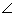MRS = 29o

Calculate: (i) ∠RNM; (ii) ∠NRM.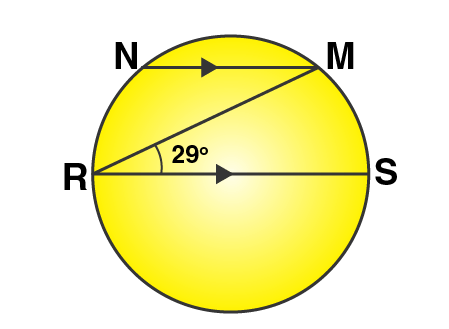Solution: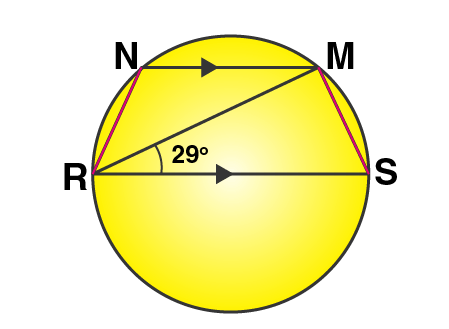(i) Join RN and MS

∠RMS = 90o [Angle in a semi-circle is a right angle]

So, by angle sum property of ∆RMS

∠RMS = 90o – 29o = 61o

And,

∠RNM = 180o – ∠RSM = 180o – 61o = 119o

[Pair of opposite angles in a cyclic quadrilateral are supplementary]

(ii) Now as RS || NM,

∠NMR = ∠MRS = 29o [Alternate angles]

∠NMS = 90o + 29o = 119o

Also, we know that

∠NRS + ∠NMS = 180o

[Pair of opposite angles in a cyclic quadrilateral are supplementary]

∠NRM + 29o + 119o = 180o

∠NRM = 180o – 148o

Therefore,

∠NRM = 32o

26. In the figure given alongside, AB || CD and O is the center of the circle. If ADC = 25o; find the angle AEB. Give reasons in support of your answer.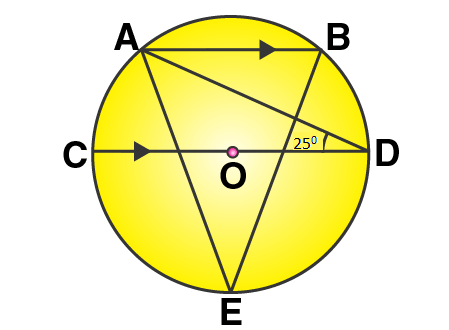Solution: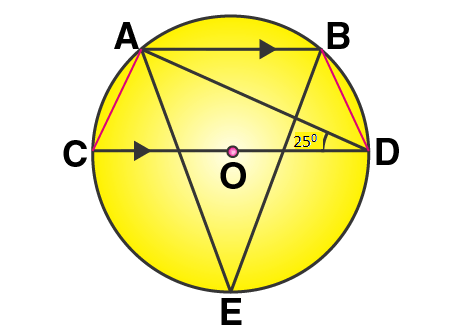Join AC and BD.

So, we have

∠CAD = 90o and ∠CBD = 90o

[Angle is a semicircle is a right angle]

And, AB || CD

Thus,

∠ADB = 180o – 25o – ∠BAC = 180o – 25o – 115o = 40o

[Pair of opposite angles in a cyclic quadrilateral are supplementary]

Finally,

[Angles subtended by the same chord on the circle are equal]

27. Two circles intersect at P and Q. Through P, a straight line APB is drawn to meet the circles in A and B. Through Q, a straight line is drawn to meet the circles at C and D. Prove that AC is parallel to BD.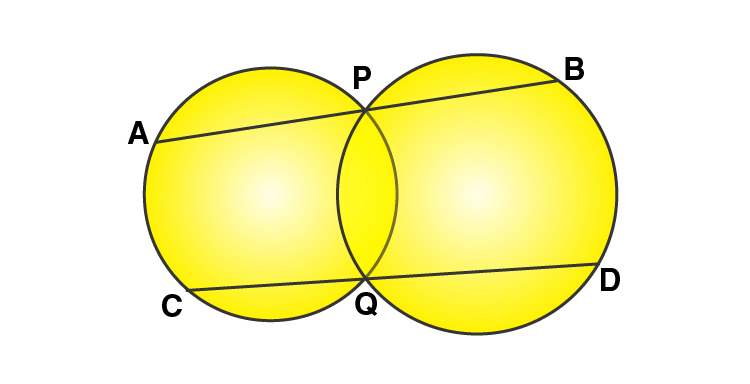Solution: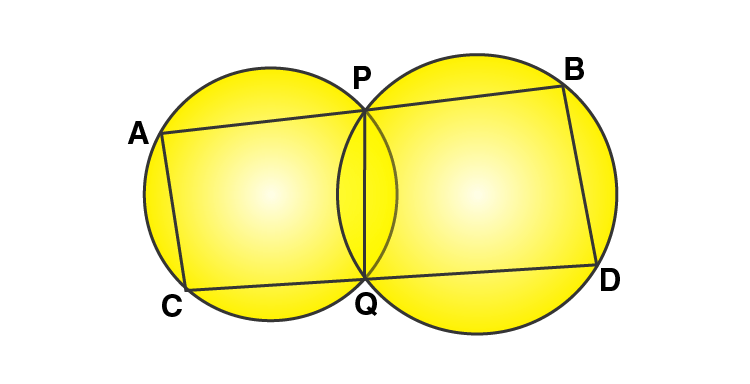Let’s join AC, PQ and BD.

As ACQP is a cyclic quadrilateral

∠CAP + ∠PQC = 180o ……. (i)

[Pair of opposite angles in a cyclic quadrilateral are supplementary]

Similarly, as PQDB is a cyclic quadrilateral

∠PQD + ∠DBP = 180o ……. (ii)

Again, ∠PQC + ∠PQD = 180o …… (iii) [Linear pair of angles]

Using (i), (ii) and (iii) we have

∠CAP + ∠DBP = 180o

Or ∠CAB + ∠DBA = 180o

We know that, if the sum of interior angles between two lines when intersected by a transversal are supplementary.

Then, AC || BD.

28. ABCD is a cyclic quadrilateral in which AB and DC on being produced, meet at P such that PA = PD. Prove that AD is parallel to BC.

Solution: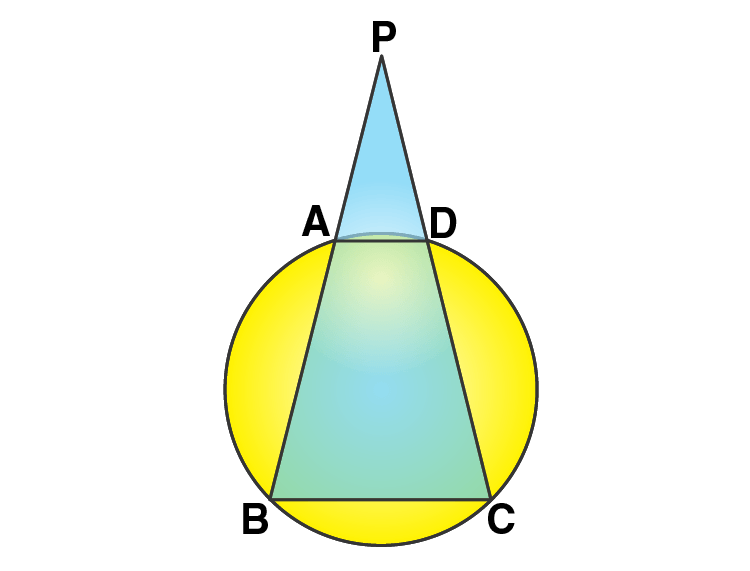Let’s assume that ABCD be the given cyclic quadrilateral.

Also, PA = PD [Given]

So, ∠PAD = ∠PDA …… (1)

[Angles opposite to equal sides are equal]

And,

Similarly,

∠CDA = 180o – ∠PDA = 180o – ∠PAD [From (1)]

As the opposite angles of a cyclic quadrilateral are supplementary,

∠ABC = 180o – ∠CDA = 180o – (180o – ∠PAD) = ∠PAD